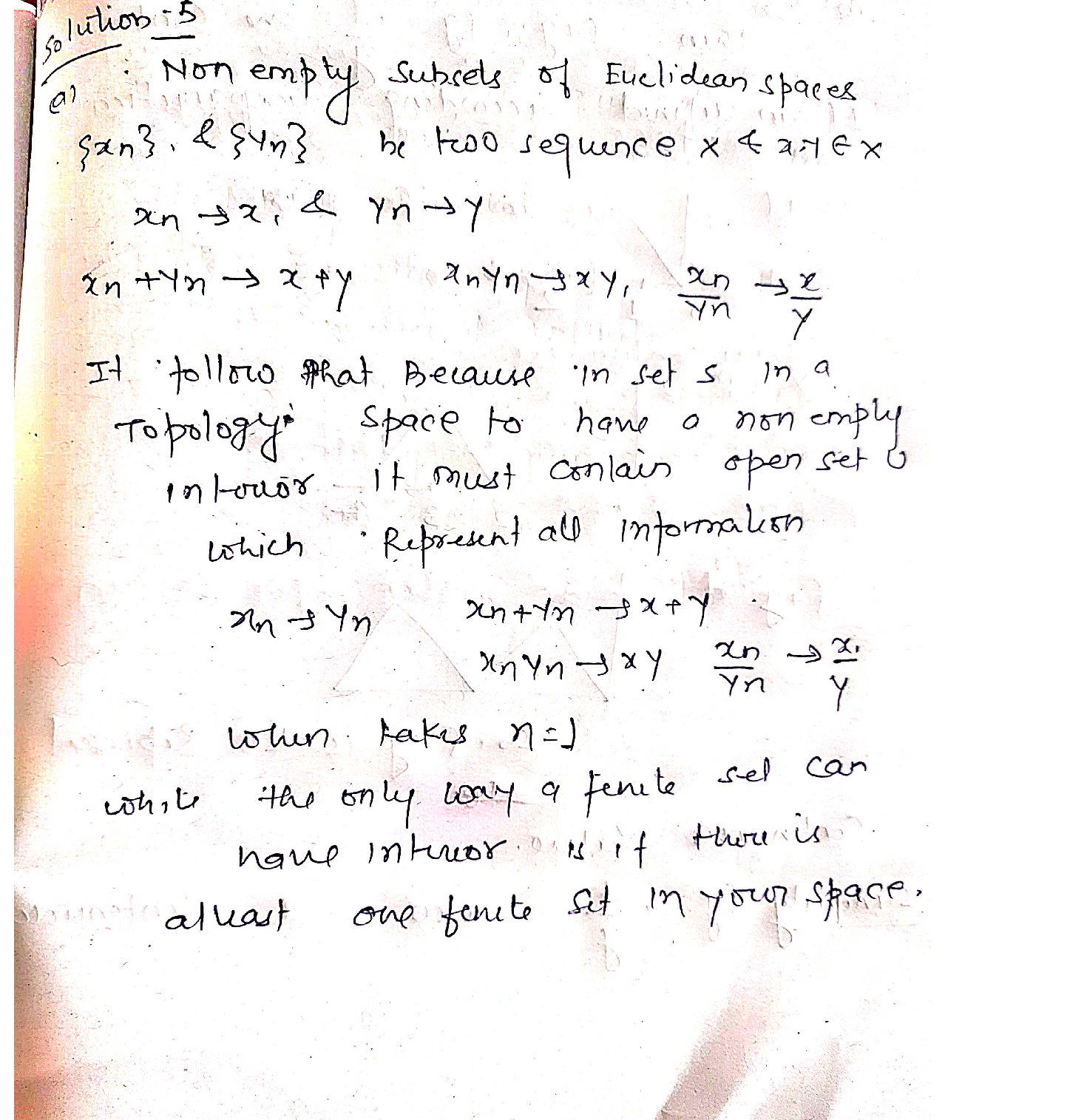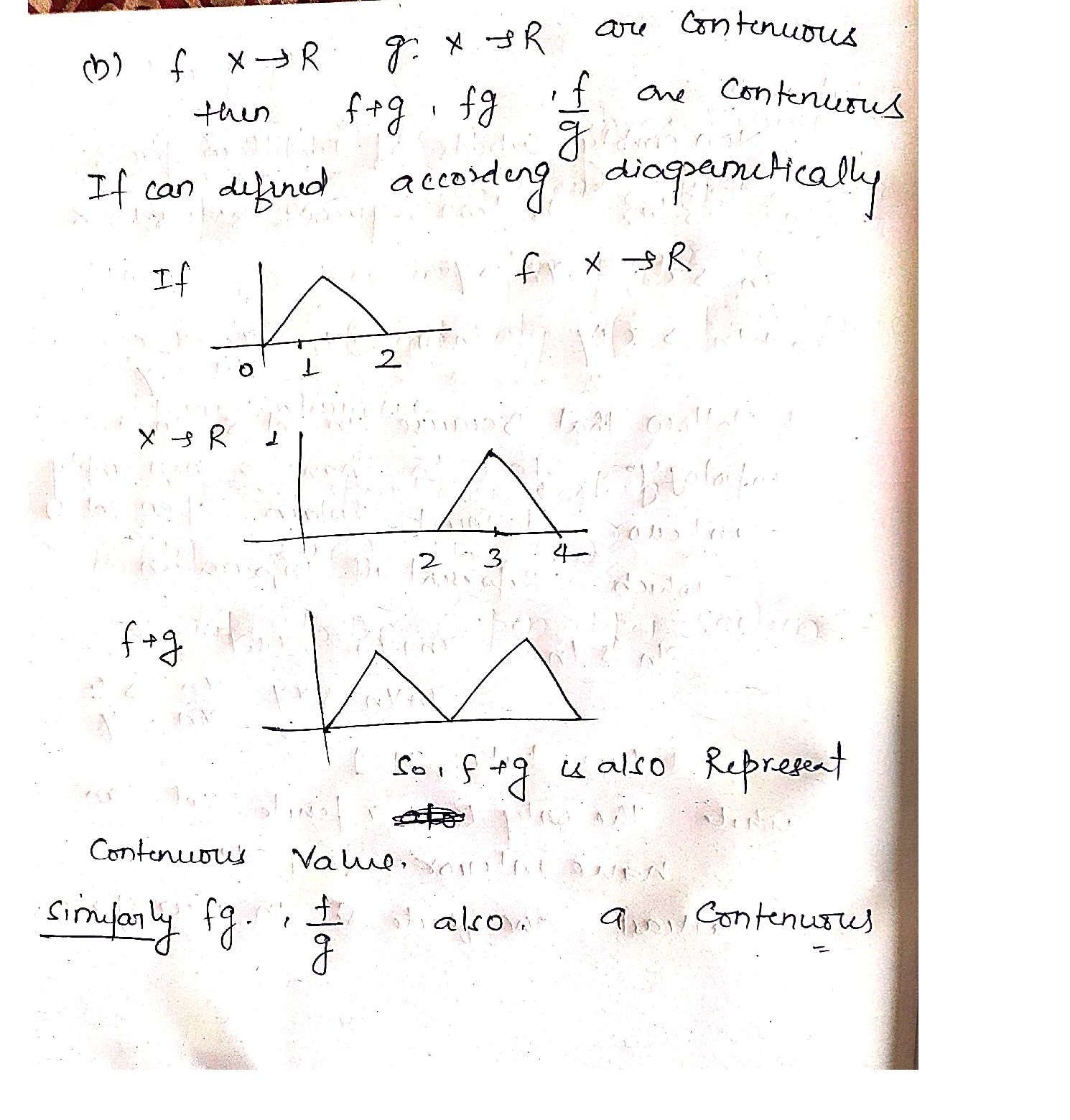Question

Numerical analysis

5. Let X,Y, Z be nonempty subsets of Euclidean spaces. [Each part is worth 10pt]Verified### Question 43428Numerical analysis

Q6. Let (X, d) and (Y, p) be metric spaces. Show that the following two definitions of lower hemicontinuity are equivalent. [Each direction is worth 10pt]

### Question 43427Numerical analysis

5. Let X,Y, Z be nonempty subsets of Euclidean spaces. [Each part is worth 10pt]

### Question 43426Numerical analysis

Prove the following statements. [Each part is worth 5pt
(a) Let {rn} be a converging sequence in a metric space X and let x € X be its limit. Use the definition of compactness to show that the set {x}U{xn} is compact.
(b) Show that a subset of a metric space X is closed if and only if its intersection with every compact subset of X is closed.

### Question 43425Numerical analysis

Let X be a non-empty set. [Each part is worth 5pt]
\text { (a) Let } d: X \times X \rightarrow \mathbf{R}_{+} \text {be defined as }
d(x, y)=\left\{\begin{array}{ll} 0 & \text { if } x=y \\ 1 & \text { if } x \neq y \end{array}\right.
Show that d is a metric on X. This metric is called discrete.
(b) Let d be a metric on X and let f : R4 → R4. If ƒ is strictly increasing, is it true that fod is a metric on X?

### Question 37438Numerical analysis

If the Taylor series for f(x) = e²+1 about x = 0 is truncated after the 10th term and is then used to compute e³, what bound on the error can be given?

### Question 31202Numerical analysis

5. In this problem, you will write a code that will find all of the roots of the function f(x) = x5 – 3x² +1 inside the interval [-2, 2]. For each part, please submit a print-out of your code along with the values you obtain for the roots.
(a) Using the fact that the roots of f on this interval are separated by at least 0.25, write a program that implements the bisection algorithm to obtain estimates of the roots that are accurate to within 01
(b) Using the estimates of the roots obtained in the previous part, write a program that implements Newton's method to refine the values of the roots such that the difference between successive iterates is at most 10-12.

### Question 31201Numerical analysis

4. [5pt] Raytracing is an algorithm that involves finding the point at which a ray (a line with a direction and an origin) intersects a curve or surface. We will consider a ray intersecting with an ellipse. The general equation for an ellipse is
\left(\frac{x}{\alpha}\right)^{2}+\left(\frac{y}{\beta}\right)^{2}-1=0
and the equation for a ray starting from the point Po = [xo, Yo] in the direction Vo = [u0, vo], is
\mathbf{R}(t)=\left[x_{0}+t u_{0}, y_{0}+t v_{0}\right]
where t e [0, x) parameterizes the ray. In this problem we will take a = 3, B = 2, Po = [0, b],Vo = [1, –0.3]. Using your favorite root finding algorithm write a code which computes theintersection of the given ray and the ellipse and plot your results. .
(a) Plug the equation for the ray, R(t), into the equation for the ellipse and analytically (with pen and paper) solve for the value of t which gives the point of intersection, call it ti.
li.(b) Perform the same calculation numerically using your favorite root finder. Report your answer to within an error of 10-6 and justify how you found the minimum number of iterations required to achieve this tolerance. Also report the point of intersection P1 = R(t1)

### Question 31200Numerical analysis

Consider the following ordinary differential equation (ODE):
\frac{d u}{d t}=g(u)
To solve this numerically, you can use the backward Euler method, for some time step At > 0
\frac{u^{n+1}-u^{n}}{\Delta t}=g\left(u^{n+1}\right)
The numerical result from this process is the sequence uº, u', u², ..., which can be interpreted as an approximation to the exact solution sampled at times 0, delta t,2 delta t, . ..
(a) If g(u) is a general nonlinear function and is differentiable, write down an iteration which determines un+1 from Newton's method.
(b) The convergence of Newton's method depends on the choice of the initial guess. What would be a sensible choice for an initial guess?

### Question 31199Numerical analysis

| In this problem, you will prove the rate of convergence for the secant method.
x_{k+1}=x_{k}-\frac{x_{k}-x_{k-1}}{f\left(x_{k}\right)-f\left(x_{k-1}\right)} f\left(x_{k}\right)
can be rewritten in the form:
x_{k+1}=\frac{x_{k} f\left(x_{k-1}\right)-x_{k-1} f\left(x_{k}\right)}{f\left(x_{k-1}\right)-f\left(x_{k}\right)}
\psi\left(x_{k}, x_{k-1}\right)=\frac{x_{k+1}-\xi}{\left(x_{k}-\xi\right)\left(x_{k-1}-\xi\right)}
where xk+1 is as in (1). Compute (for fixed value of xk-1)
\varphi\left(x_{k-1}\right)=\lim _{x_{k} \rightarrow \xi} \psi\left(x_{k}, x_{k-1}\right)
(c) Now compute
\lim _{x_{E-1} \rightarrow \xi} \varphi\left(x_{k-1}\right)
and therefore show thatlim
\lim _{x_{k}, x_{k-1} \rightarrow \xi} \psi\left(x_{k}, x_{k-1}\right)=\frac{f^{\prime \prime}(\xi)}{2 f^{\prime}(\xi)}
(d) Next, assume that the secant method has convergence order q, that is to say that
\lim _{k \rightarrow \infty} \frac{\left|x_{k+1}-\xi\right|}{\left|x_{k}-\xi\right|^{q}}=A<\infty
Using the above results, show that q – 1– 1/q = 0, and therefore that q = (1+ v5)/2.
(e) Finally, show that this implies that
\lim _{k \rightarrow \infty} \frac{\left|x_{k+1}-\xi\right|}{\left|x_{k}-\xi\right|^{q}}=\left(\frac{f^{\prime \prime}(\xi)}{2 f^{\prime}(\xi)}\right)^{q /(1+q)}

### Question 30120Numerical analysis

Perform three iterations of the Modified Secant method starting with the initial guess x0=0.6 and using the increment 0.001.

### Submit query